Jr Math section of MathBitsNotebook.comFactoringTopics Standards: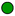NGMS-6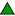CCSS 6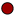NGMS-7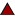CCSS 7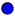NGMS-8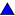CCSS 8

Divisibility Rules (table of possible rules)
Chart
Prime Factorization (primes, Fund. Thm of Arithmetic, examples)
Review
*
Common Factors (w/ GCF, LCM, listing, distributive sum, prime factor diagram)Factoring - Numerical Examples (factor, primes, sums, geometry)Sieve of Eratosthenes (for finding prime numbers)``
Extra
NOTE: Find "factoring linear expressions" under "Algebraic Expressions"

 PRACTICE: • Practice with Prime Factorization Review • Practice with Common Factors• Practice Factoring• Practice Factoring Word Problems• Vocabulary - Word Guess Game (topical vocabulary game - iPad compatible)